# Six Sigma DMAIC Process - Measure Phase - Data Collection Strategy - Sampling

Sampling is the process of selecting a small number of elements from a larger defined target group of elements. Population is the total group of elements we want to study. Sample is the subgroup of the population we actually study. Sample would mean a group of ‘n’ employees chosen randomly from organization of population "N". Sampling is done in situations like:
• We sample when the process involves destructive testing, e.g. taste tests, car crash tests, etc.
• We sample when there are constraints of time and costs
• We sample when the populations cannot be easily captured
Sampling is NOT done in situations like:
• We cannot sample when the events and products are unique and cannot be replicable
Sampling can be done by following methods:
Probability Sampling:
• Simple Random Sampling
• Stratified Random Sampling
• Systematic Sampling
• Cluster Sampling
Non Probability Sampling:
• Convenience Sampling
• Judgment Sampling
• Quota Sampling
• Snowball Sampling
Simple Random Sampling:
Simple random sampling is a method of sampling in which every unit has equal chance of being selected.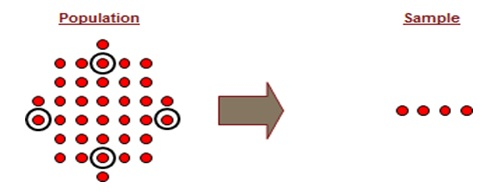Six Sigma Simple Random Sampling

Stratified Random Sampling:
Stratified random sampling is a method of sampling in which stratum/groups are created and then units are picked randomly.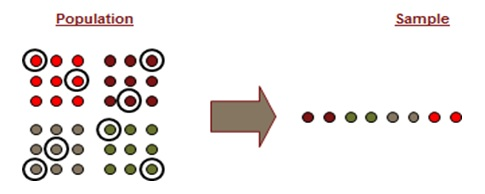Six Sigma Stratified Random Sampling

Systematic Sampling:
Systematic sampling is a method of sampling in which every nth unit is selected from the population.Six Sigma Systematic Sampling

Cluster Sampling:
Cluster sampling is a method of sampling in which clusters are sampled every Tth time.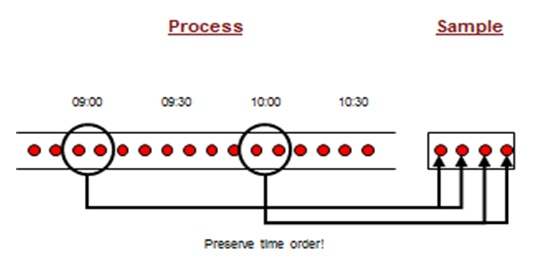Six Sigma Cluster Sampling

Non-Probability Sampling:
Convenience sampling relies upon convenience and access.
Judgment sampling relies upon belief that participants fit characteristics.
Quota sampling emphasizes representation of specific characteristics.
Snowball sampling relies upon respondent referrals of others with like characteristics.

Sampling Bias:
Bias occurs when systematic differences are introduced into the sample as a result of the selection process. A sample that is biased will not be representative of the population. A sample that is biased will lead to incorrect conclusions about the population. The types of sampling bias are as follows:
• Convenience sampling selection bias: Occurs when the sample is drawn only from the part of the population that is easily accessible
• Systematic sampling selection bias: Can introduce a bias if the procedure matches an underlying structure
• Environmental bias: Introduced when environmental conditions have changes from the time the sample was drawn to the time the sample is used to draw conclusions about the population
• Non-response bias: Initiated by respondents. Only a subset of the population responds to the survey
Sample Size Formula:
In order to determine the sample size, we need to identify if the data type is continuous or discrete, whether we have standard deviation or proportion defectives and the confidence level.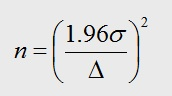Six Sigma Sample Size Formula – Continuous Data

Here, n = Sample Size, σ = is the estimated standard deviation of our population and
Δ – is the precision or the level of uncertainty in your estimate that you are willing to accept (expressed in %).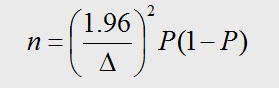Six Sigma Sample Size Formula – Discrete Data

Where, P – is the proportion defective that we are estimating (expressed in %) and
Δ – is the precision or the level of uncertainty in your estimate that you are willing to accept (expressed in %).

Let us solve a few questions to understand the formula better.
Given a sample size of 100, how precisely can we estimate a proportion defective estimated as P = 20%?
Here, P = 20% and n = 100, we need to find Δ.
Using the formula for Sample Size – Discrete Data,
Δ2 = (n)/ (1.96)2 * P(1 – P)
Δ2 = 100 / (3.8416) * 0.16
Δ2 = 162.681
Δ = 12.75

Given an estimated proportion defective guessed to be somewhere in the range of 5% to 15%, how many observations should we take to estimate the proportion defective within 2%?
Here, P = (15% - 5%) = 10% = 0.10, Δ = 0.02
Using the formula for Sample Size – Discrete Data,
n = (1.96/0.02)2 * (0.10)*(1-0.10)
n = 9604 * 0.09
n = 864.36

We want to estimate the average cycle time within 2 days. A preliminary estimate of the population standard deviation is 8 days. How many observations should we take?
Here, Δ = 2 and σ = 8 days
Using the formula for Sample Size – Continuous Data,
n = (1.96*8/2)2
n = 61.47

# We guarantee that Your Free Online Training will make you pass Your Six Sigma Certification Exam!